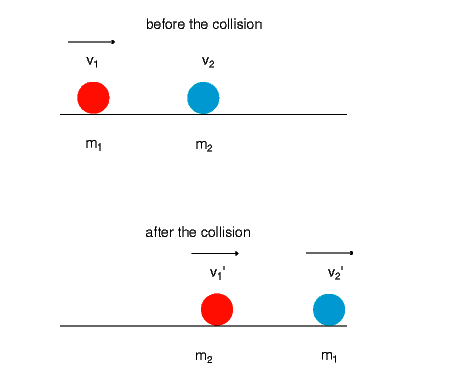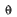# Collisions

There are 2 kinds of collisions: inelastic and elastic .

Inelastic collisions are collisions in which the objects remain attached after the collision.

(For example, if you placed a glob of glue on the front of a billiard ball, then had that ball and another ball collide, they would stick together at the moment of impact.) If the objects have equal masses, the total momentum of the system before and after the collision must be equal to zero. (Remember, momentum is vector, so one direction is positive and one is negative.) When objects of equal and opposite momenta (plural of momentum) collide inelastically, they come to rest. (EK is not conserved, but taking all forms of energy into consideration, including heat, energy and momentum are conserved.)

Elastic collisions occur when colliding objects rebound from each other without a loss of. (Actually, the only perfectly elastic collisions occur between atomic and subatomic particles because of the loss of someas heat.) This is true for objects in 1-dimensional and 2-dimensional collisions. If you consider a situation in which 2 billiard balls (A & B) collide at an angle, you can see this:

This means that when 2 objects of equal mass collide at an angle other than head-on in an elastic collision, they will move away from each other at right angles.(NOTE: A and B represent motion before collision; A’ and B’ represent motion after collision.)

Remember that for an inelastic collision, the mass afterward includes both the before masses (they stick together).

## Elastic CollisionsElastic collisions mean that the two objects literally bounce off each other. Momentum is always conserved! If this is a perfectly elastic collision, then the kinetic energy is also conserved.

m1v1 + m2v2 = m1v1f + m2v2f

K1i + K2i = K1f + K2f (if 100% elastic)

Collisions between molecules in an ideal gas are considered to be perfectly elastic…you may have studied that in Chemistry!

## Inelastic CollisionsIn an inelastic collision, the objects stick to each other and move off as one entity after collision. Momentum is always conserved! Kinetic energy is not conserved…some is lost to friction, heat of deformation, etc. because of the process of sticking together.

m1v1 + m2v2 = (m1 + m2)vf

## Recoil

Recoil is a special case of an elastic collision. If the two objects either start at rest or end at rest, then it is a recoil situation. For example, if two skaters are standing on a frozen pond facing each other and push against each other, they will each move backward with the same momentum. Their momenta are equal, but in opposite directions. The final momentum has to equal the initial momentum (of zero) to obey the law of conservation of momentum. Therefore,

m1v1 = -m2v2

A rifle example shows the principle of recoil. Both start at rest, so the momentum of the bullet forward must be equal and opposite to the momentum of the rifle backwards.

A rocket taking off also illustrates this principle. The rocket’s momentum upward must equal the momentum of the fuel downward.

If two football players are running toward each other and meet and fall down motionless, then the momentum of each before collision must have perfectly balanced to make the momentum after collision equal to zero! Do remember that momentum is a vector and that direction is very important!

Sample 2-D Momentum Problem

Two cars meet unfortunately at an intersection with the following data:

Car 1, mass 3000. kg is traveling at 20.0 m/s at 25 degrees North of East. Car 2, mass of 4000. kg, is traveling at 25.0 m/s at 75 degrees north of west.

Find the magnitude and direction of the velocity of the combined wreckage.

This is a vector problem…momentum is a vector, so draw a free-body diagram to illustrate the velocities of the two cars.

v2=25 m/s v1=20.0 m/s2 =105o1=25o

This is an inelastic collision problem..use m1v1 + m2v2 = (m1 + m2) vf

The law of conservation of momentum is applied in each axis separately.

Vfx : (m1v1x + m2v2x) / (m1 + m2) =

[(3000 kg)(20 m/s)(cos 25) + (4000 kg)(25 m/s) (cos 105)] / 7000 kg = 4.07 m/s

Vfy : (m1v1y + m2v2y) / (m1 + m2) =

[(3000 kg)(20 m/s)(sin 25) + (4000 kg)(25 m/s) (sin 105)] / 7000 kg = 17.4 m/s

V = sq rt (vfx 2 + vfy 2) =

Sq rt [(4.07 m/s)2 + (17.4 m/s)2]= 17.9 m/s= tan-1 (y/x) = tan -1 (17.4/4.07) = 76.8o

Magnitude = 17.9 m/s, direction is 76.8o

(source)